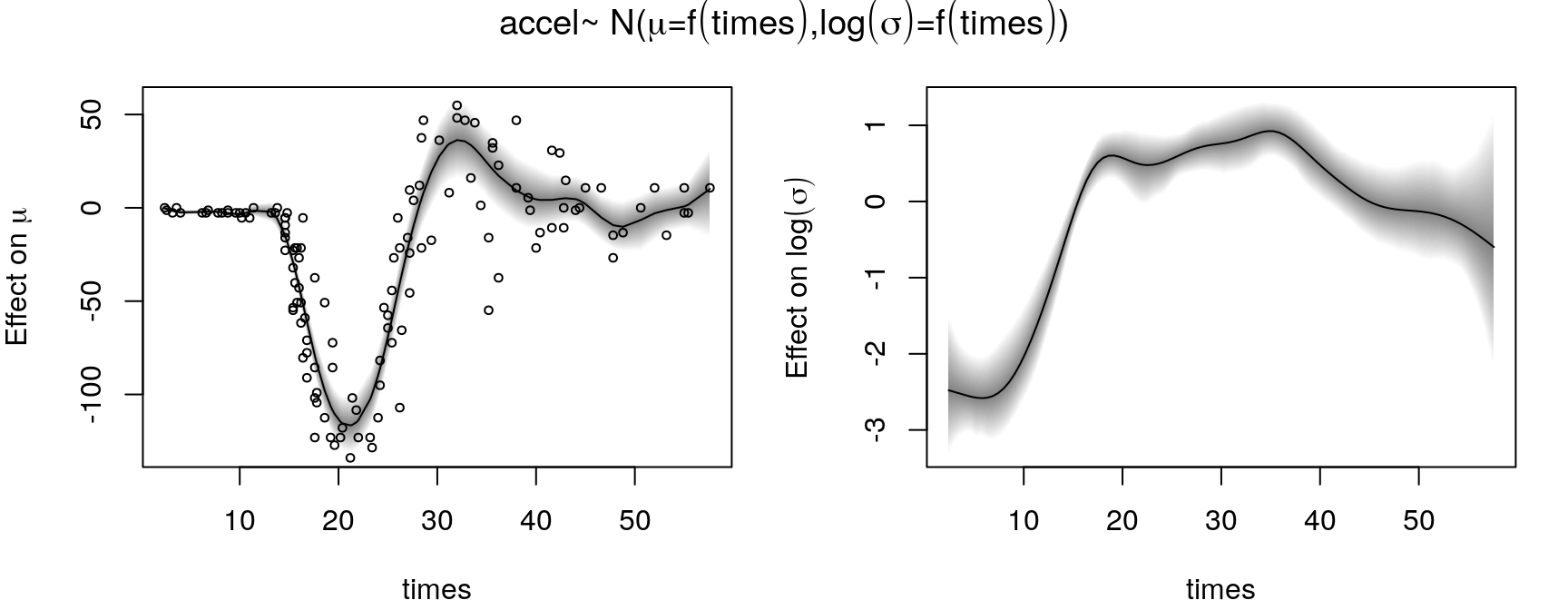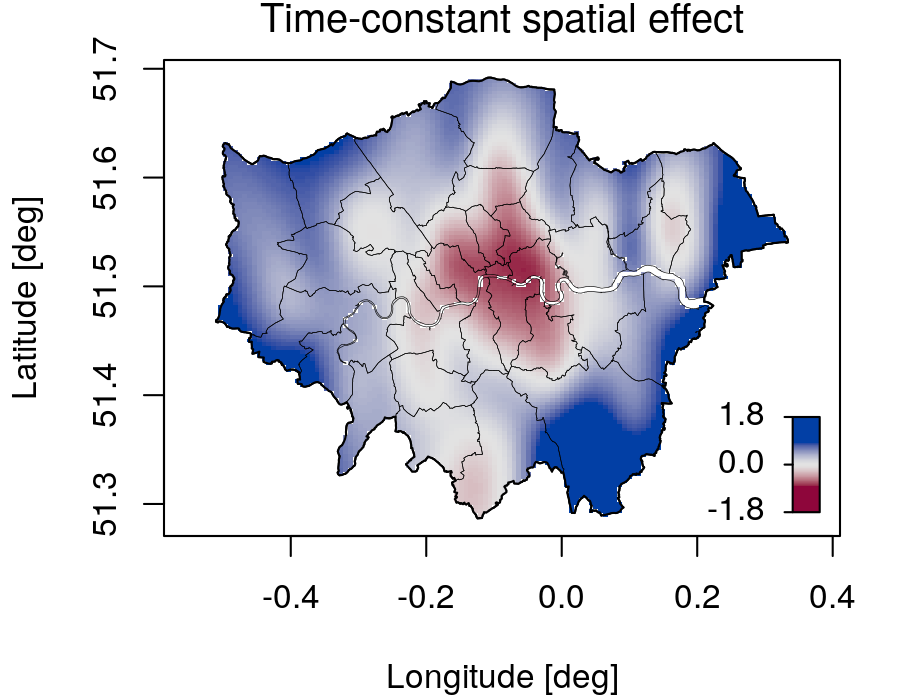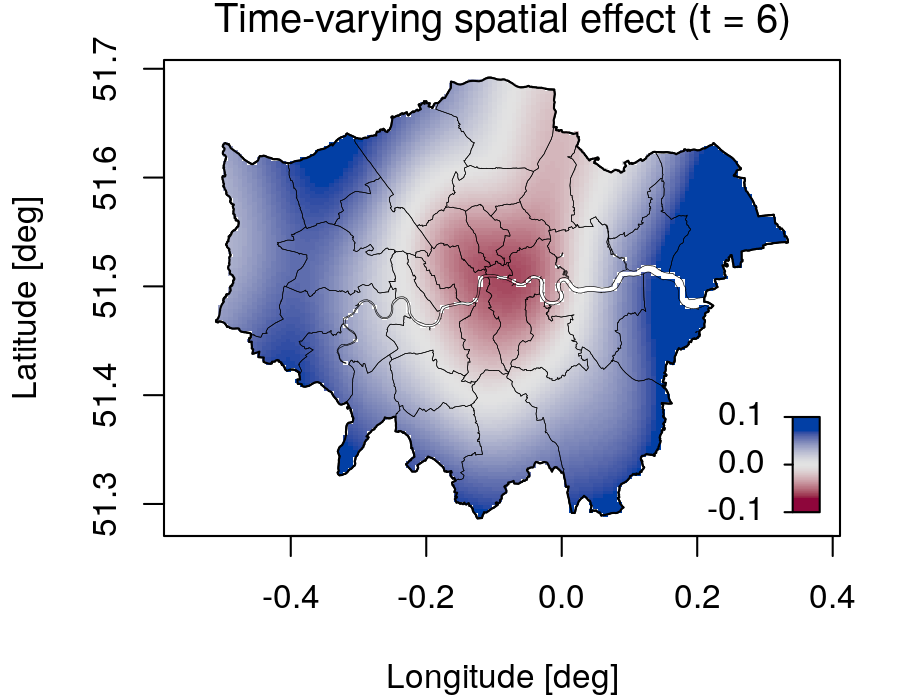A Lego Toolbox for Flexible Bayesian Regression (and Beyond)

# A Lego Toolbox for Flexible Bayesian Regression (and Beyond)

The bamlss package provides a modular computational framework for flexible Bayesian regression models (and beyond). Users can specify complex models using the familiar formula syntax of R. The package supports commonly used extractor functions like plot(), summary(), predict(), etc. Moreover, users can specify their own model term constructors or even model fitting engines, Bayesian or frequentist.

## Flexible model terms## Spatial effects